Related Articles

# Split-apply-combine strategy on DataFrames in Julia

• Last Updated : 01 Aug, 2020

Julia is a high performance, dynamic programming language that has a high-level syntax. It might also be considered as a new and easier variant of python language. Data frames can be created, manipulated, and visualized in various ways for data science and machine learning purposes with Julia.

#### Split-Apply-Combine Strategy

For some tasks in data analysis, splitting data frames is required to apply multiple functions, and the final results are combined. We have to access the necessary packages and can use the by or the aggregate function to implement this strategy.

First, we have to add the necessary packages to use DataFrames, CSV files, and required functions.

## Julia

 `# Adding Packages for using DataFrames, ``# CSV files, and statistical functions``using Pkg``Pkg.add(``"DataFrames"``)``Pkg.add(``"CSV"``)``Pkg.add(``"Statistics"``)`

Now we read a CSV file into a DataFrame. A dataset of video game sales information, located in the local memory is being used here.

## Julia

 `# Enabling use of necessary packages``using DataFrames, CSV, Statistics`` ` `# Reading a CSV file into a DataFrame``ds ``=` `CSV.read(``"C:\\Users\\metal\\vgsales.csv"``);`

This dataframe is now split into two parts with the use of pre-defined functions head() and tail().

## Julia

 `# Displaying the first few rows of the DataFrame``head(ds)`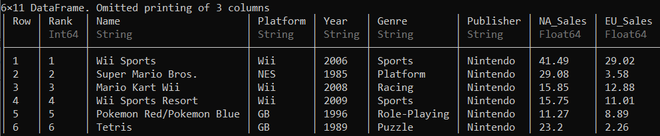## Julia

 `# Displaying the last few rows of the DataFrame``tail(ds)`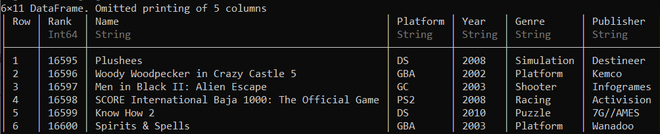Now, we use the by function and the three arguments that can be passed in the function are:

1. DataFrame
2. Columns to split the DataFrame on
3. Functions to be applied after splitting of the DataFrame

Now the DataFrame is split on a column and we will perform various functions on it.

## Julia

 `# Calcuating the number of rows and columns ``# for each of the publishers in the DataFrame``by(ds, :Publisher, size)`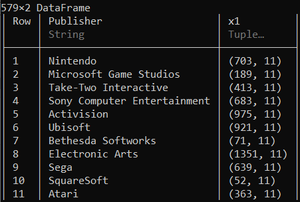## Julia

 `# Calcuating the mean of the global sales of each Publisher``by(ds, :Publisher, df ``-``> mean(df.Global_Sales))`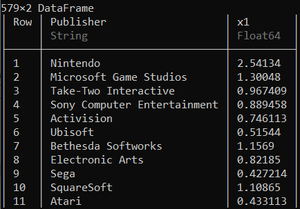## Julia

 `# Calculating number of entries for each publisher``by(ds, :Publisher, df ``-``> DataFrame(N ``=` `size(df, ``1``)))`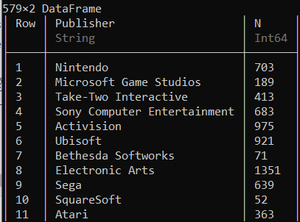We can also place the functions and expressions in a do block as shown below:

## Julia

 `# Calculating the mean and variance ``# of global sales of each publisher``by(ds, :Publisher) do df``  ``DataFrame(Mean ``=` `mean(df.Global_Sales), Variance ``=` `var(df.Global_Sales))``end`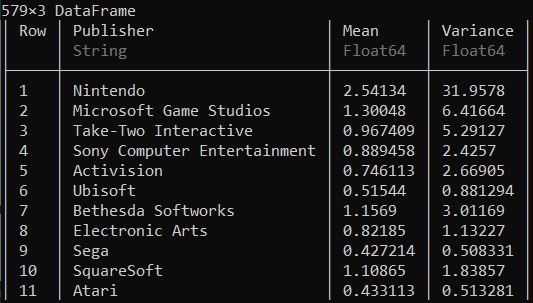As mentioned, the aggregate() function can also be used to implement the strategy, which takes in the same three arguments as the by() function. After passing the arguments with a specific function, it creates new columns as a result, named with the syntax ‘column.name_function’.

## Julia

 `# Calculating the number of entries ``# in each column for each publisher``aggregate(ds, :Publisher, length)`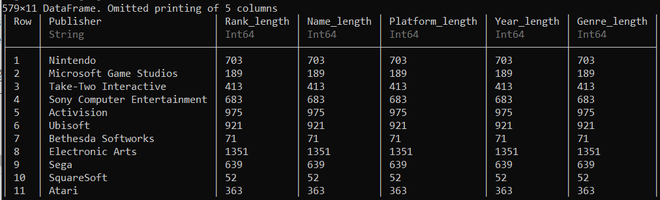We can also create subsets by splitting the dataset using the groupby() function

## Julia

 `# Creating a subset of entries for each publisher``for` `subdf ``in` `groupby(ds, :Publisher)``   ``println(size(subdf, ``1``))``end`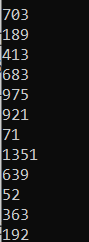Various other functions can be passed as arguments for the by() and the aggregate() functions to implement the Split-Apply-Combine strategy to achieve the desired results and insights.

My Personal Notes arrow_drop_up